### Home > CALC > Chapter 7 > Lesson 7.2.1 > Problem7-65

7-65.
1.No calculator! Given f(x) = 3x − 2 and g(x) =sinx, find the following limits: Homework Help ✎

1.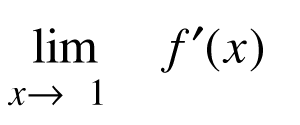2.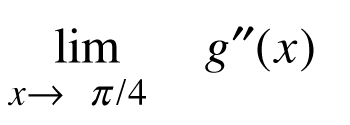3.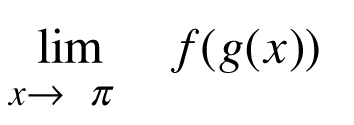4.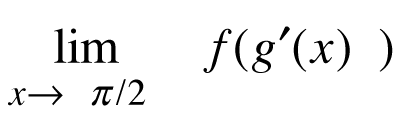5.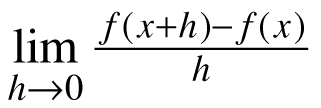6.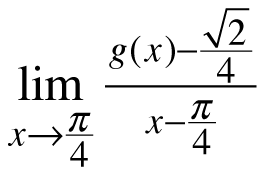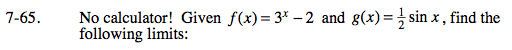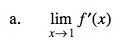$\frac{d}{dx}a^{x}=\text{ln}x\cdot a^{x}$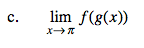$\lim_{x\to\pi}(3^{0.5\sin(x)}-2)$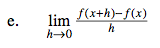This is Hana's definition of the derivative.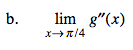$\frac{d}{dx}\text{sin}x=\text{cos}x; \frac{d}{dx}\text{cos}x=-\text{sin}x$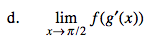Be mindful about positive and negative values.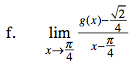This is Ana's definition of the derivative.

$g'\left ( \frac{\pi }{4} \right )={\_\_\_\_\_}$